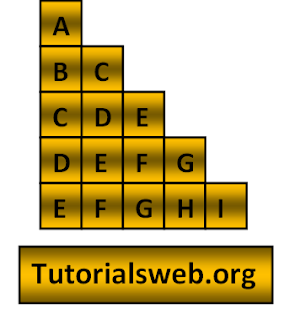##To understand this example, you should have knowledge of the following topics:

·   Java programming operators.

Java Character pattern5 program using For loop:
`import java.util.Scanner;`
`class alphabetPattern5`
`{`
`public static void main(String[] ags)`
`{`
`    int i, j,k,n;`
`   System.out.println("Enter the number of lines");`
`   Scanner s = new Scanner(System.in);`
`   n = s.nextInt();`
`    for(i = 1;i <= n;i++)`
`    { `
`        k = i;`
`        for(j = 1;j <= i;j++,k++)`
`        {`
`            System.out.print((char)(k+64));`
`        }`
`        System.out.println();`
`    }`
`  }`
Output:
```
Enter the number of lines
5
A
BC
CDE
DEFG
EFGHI
```
Explanation:
• First four integers "i", "j", "k" and "n" are declared of type int.
• Then in the First for loop “i” value is initialized with a value 1.
• Now “i” value is checked with the condition i < n that is (where n value is given through Keyboard). If the condition is True loops enter into second for loop.
• Now the loop enters into second for loop and checks the condition j <= i. Where "j = 1". and the condition is True.
• Now prints the " Alphabet". Print up to second for loop condition becomes False.
• When the value of "i"  becomes greater than"n" value the program terminates.

## Search This Blog

### C Variables and Types of Variables in C

C variable is a data name that may be used to store a data value. The value of the C variable may get change in the program and it can be r...